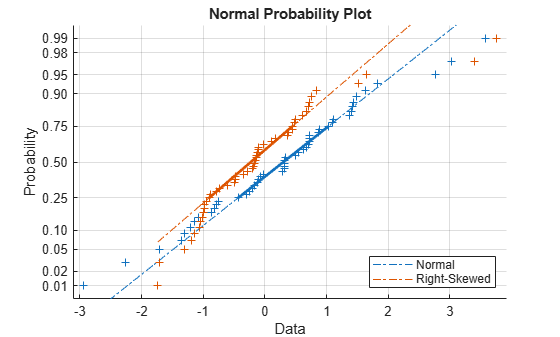Documentación

Esta página aún no se ha traducido para esta versión. Puede ver la versión más reciente de esta página en inglés.

# normplot

Normal probability plot

## Sintaxis

``normplot(x)``
``normplot(ax,x)``
``h = normplot(___)``

## Descripción

ejemplo

````normplot(x)` creates a normal probability plot comparing the distribution of the data in `x` to the normal distribution. `normplot` plots each data point in `x` using plus sign (`'+'`) markers and draws two reference lines that represent the theoretical distribution. A solid reference line connects the first and third quartiles of the data, and a dashed reference line extends the solid line to the ends of the data. If the sample data has a normal distribution, then the data points appear along the reference line. A distribution other than normal introduces curvature in the data plot.```
````normplot(ax,x)` adds a normal probability plot into the axes specified by `ax`.```

ejemplo

````h = normplot(___)` returns graphics handles corresponding to the plotted lines, using any of the previous syntaxes.```

## Ejemplos

contraer todo

Generate random sample data from a normal distribution with `mu = 10` and `sigma = 1`.

```rng default; % For reproducibility x = normrnd(10,1,25,1);```

Create a normal probability plot of the sample data.

```figure; normplot(x)```The plot indicates that the data follows a normal distribution.

Generate 50 random numbers from each of four different distributions: A standard normal distribution; a Student's-t distribution with five degrees of freedom (a "fat-tailed" distribution); a set of Pearson random numbers with `mu` equal to 0, `sigma` equal to 1, skewness equal to 0.5, and kurtosis equal to 3 (a "right-skewed" distribution); and a set of Pearson random numbers with `mu` equal to 0, `sigma` equal to 1, skewness equal to -0.5, and kurtosis equal to 3 (a "left-skewed" distribution).

```rng(11) % For reproducibility x1 = normrnd(0,1,[50,1]); x2 = trnd(5,[50,1]); x3 = pearsrnd(0,1,0.5,3,[50,1]); x4 = pearsrnd(0,1,-0.5,3,[50,1]);```

Plot four histograms on the same figure for a visual comparison of the pdf of each distribution.

```figure subplot(2,2,1) histogram(x1,10) title('Normal') axis([-4,4,0,15]) subplot(2,2,2) histogram(x2,10) title('Fat Tails') axis([-4,4,0,15]) subplot(2,2,3) histogram(x3,10) title('Right-Skewed') axis([-4,4,0,15]) subplot(2,2,4) histogram(x4,10) title('Left-Skewed') axis([-4,4,0,15])```The histograms show how each sample differs from the normal distribution.

Create a normal probability plot for each sample.

```figure subplot(2,2,1) normplot(x1) title('Normal') subplot(2,2,2) normplot(x2) title('Fat Tails') subplot(2,2,3) normplot(x3) title('Right-Skewed') subplot(2,2,4) normplot(x4) title('Left-Skewed')```Create a 50-by-2 matrix containing 50 random numbers from each of two different distributions: A standard normal distribution in column 1, and a set of Pearson random numbers with `mu` equal to 0, `sigma` equal to 1, skewness equal to 0.5, and kurtosis equal to 3 (a "right-skewed" distribution) in column 2.

```rng default % For reproducibility x = [normrnd(0,1,[50,1]) pearsrnd(0,1,0.5,3,[50,1])];```

Create a normal probability plot for both samples on the same figure. Return the plot line graphic handles.

```figure h = normplot(x)```
```h = 6x1 Line array: Line Line Line Line Line Line ```
`legend({'Normal','Right-Skewed'},'Location','southeast')`The handles h(1) and h(2) correspond to the data points for the normal and skewed distributions, respectively. The handles h(3) and h(4) correspond to the second and third quartile line fit to the sample data. The handles h(5) and h(6) correspond to the extrapolated line that extends to the minimum and maximum of each set of sample data.

To illustrate, increase the line width of the second and third quartile line for the normally distributed data sample (represented by h(3)) to 2.

```h(3).LineWidth = 2; h(4).LineWidth = 2;```## Argumentos de entrada

contraer todo

Sample data, specified as a numeric vector or numeric matrix. `normplot` displays each value in `x` using the symbol `'+'`. If `x` is a matrix, then `normplot` displays a separate line for each column of `x`.

Tipos de datos: `single` | `double`

Target axes, specified as an `Axes` object or a `UIAxes` object. `normplot` adds an additional plot into the axes specified by `ax`. For details, see Axes Properties and UIAxes Properties.

Use `gca` to return the current axes for the current figure.

## Output Arguments

contraer todo

Graphics handles for line objects, returned as a vector of `Line` graphics handles. Graphics handles are unique identifiers that you can use to query and modify the properties of a specific line on the plot. For each column of `x`, `normplot` returns three handles:

• The line representing the data points. `normplot` represents each data point in `x` using plus sign (`'+'`) markers.

• The line joining the first and third quartiles of each column of `x`, represented as a solid line.

• The extrapolation of the quartile line, extended to the minimum and maximum values of `x`, represented as a dashed line.

To view and set properties of line objects, use dot notation. For information on using dot notation, see Acceder a valores de propiedad (MATLAB). For information on the `Line` properties that you can set, see Line Properties.

## Algoritmos

`normplot` matches the quantiles of sample data to the quantiles of a normal distribution. The sample data is sorted and plotted on the x-axis. The y-axis represents the quantiles of the normal distribution, converted into probability values. Therefore, the y-axis scaling is not linear.

Where the x-axis value is the ith sorted value from a sample of size N, the y-axis value is the midpoint between evaluation points of the empirical cumulative distribution function of the data. The midpoint is equal to $\frac{\left(i-0.5\right)}{N}$.

`normplot` superimposes a reference line to assess the linearity of the plot. The line goes through the first and third quartiles of the data.

## Alternative Functionality

You can use the `probplot` function to create a probability plot. The `probplot` function enables you to indicate censored data and specify the distribution for a probability plot.

Download ebook